keras_keras 安装 - CSDN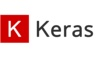keras 订阅
Keras是一个由Python编写的开源人工神经网络库，可以作为Tensorflow、Microsoft-CNTK和Theano的高阶应用程序接口，进行深度学习模型的设计、调试、评估、应用和可视化   。Keras在代码结构上由面向对象方法编写，完全模块化并具有可扩展性，其运行机制和说明文档有将用户体验和使用难度纳入考虑，并试图简化复杂算法的实现难度   。Keras支持现代人工智能领域的主流算法，包括前馈结构和递归结构的神经网络，也可以通过封装参与构建统计学习模型   。在硬件和开发环境方面，Keras支持多操作系统下的多GPU并行计算，可以根据后台设置转化为Tensorflow、Microsoft-CNTK等系统下的组件   。Keras的主要开发者是谷歌工程师François Chollet，此外其GitHub项目页面包含6名主要维护者和超过800名直接贡献者   。Keras在其正式版本公开后，除部分预编译模型外，按MIT许可证开放源代码   。 展开全文Keras是一个由Python编写的开源人工神经网络库，可以作为Tensorflow、Microsoft-CNTK和Theano的高阶应用程序接口，进行深度学习模型的设计、调试、评估、应用和可视化   。Keras在代码结构上由面向对象方法编写，完全模块化并具有可扩展性，其运行机制和说明文档有将用户体验和使用难度纳入考虑，并试图简化复杂算法的实现难度   。Keras支持现代人工智能领域的主流算法，包括前馈结构和递归结构的神经网络，也可以通过封装参与构建统计学习模型   。在硬件和开发环境方面，Keras支持多操作系统下的多GPU并行计算，可以根据后台设置转化为Tensorflow、Microsoft-CNTK等系统下的组件   。Keras的主要开发者是谷歌工程师François Chollet，此外其GitHub项目页面包含6名主要维护者和超过800名直接贡献者   。Keras在其正式版本公开后，除部分预编译模型外，按MIT许可证开放源代码   。

François Chollet 等 

Linux，macOS，Windows

0.1.0/2015年6月13日 

Keras

Python

2.3.0/2019年09月17日 
Keras历史与命名
Keras的前身是François Chollet为ONEIROS（Open-ended Neuro-Electronic Intelligent Robot Operating System）项目所的编写的代码，在2015年分离成为开源的人工神经网络工具。Keras的最初版本以Theano为后台，设计理念参考了Torch但完全由Python编写   。2015年11月的Keras测试版本0.3.0，Tensorflow被加入了后台选项。2016年4月，Keras在PIPy上发布了第一个稳定版本1.0.0   。2017年5月，Keras版本2.0.0发布   。同年6月，Keras版本2.0.5测试性地将Microsoft-CNTK加入后台选项   。自2017年起，Keras得到了Tensorflow团队的支持，其大部分组件被整合至Tensorflow的Python API中。在2018年Tensorflow 2.0.0公开后，Keras被正式确立为Tensorflow高阶API，即tf.keras   。此外自2017年7月开始，Keras也得到了CNTK 2.0的后台支持   。在2019年9月17日Keras稳定版本2.3.0的更新文档中，Keras团队宣布了计划调整：在该版本之后，Keras将集中于Tensoflow后台的有关内容，多后台Keras的开发优先度被降低，且更新将仅包含补丁（bug fix），不会有新的功能性内容移植/加入   。Keras的命名来自古希腊语“κέρας （牛角）”或 “κραίνω（实现）”，意为将梦境化为现实的“牛角之门”   。由荷马史诗《奥德赛》第19卷佩涅罗佩与奥德修斯的对话，无法挽留的梦幻拥有两座门，一座门由牛角制成，一座门由象牙制成，象牙之门内光彩夺目，却仅是无法实现的梦境；唯有走进牛角之门，才能由梦境看见真实   。

• 通过大量实战，掌握Tensorflow和Keras经常用到的各种建模方式，参数优化方法，自定义参数和模型的手段，以及对训练结果评估与分析的技巧。 2 从机器学习基础算法开始，然后进入到图像分类领域，使用MNIST...深度学习 深度学习框架 深度学习算法 python深度学习 深度强化学习
• ## keras 入门上

千次阅读 多人点赞 2018-04-26 17:55:17
1. 什么是 KerasKeras 是基于 Theano 或 TensorFlow 的一个深度学习...安装 Keras使用 Keras 前还需要安装 Numpy、Scipy 等 Python 包，建议直接安装 Python 科学计算环境 Anaconda，一步到位。然后直接通过 pip...
1. 什么是 KerasKeras 是基于 Theano 或 TensorFlow 的一个深度学习框架，它的设计参考了 Torch，用 Python 语言编写，是一个高度模块化的神经网络库，支持 GPU 和 CPU。安装 Keras使用 Keras 前还需要安装 Numpy、Scipy 等 Python 包，建议直接安装 Python 科学计算环境 Anaconda，一步到位。然后直接通过 pip install keras 安装 Keras 就可以了，非常的方便。在 Theano 和 TensorFlow 间切换Keras 的底层库使用 Theano 或 TensorFlow，这两个库也称为 Keras 的后端。无论是 Theano 还是 TensorFlow，都是一个“符号式”的库，这也使得 Keras 的编程与传统的 Python 代码有所差别。笼统的说，符号主义的计算首先定义各种变量，然后建立一个“计算图”，计算图规定了各个变量之间的计算关系。建立好的计算图需要编译以确定其内部细节，但是此时的计算图只是一个“空壳子”，里面没有任何实际的数据，只有当你把需要运算的输入放进去后，才能在整个模型中形成数据流，从而形成输出值。Keras 的模型搭建形式就是这种方法，搭建好的 Keras 模型只是一个空壳子，只有实际生成可调用的函数后（K.function），输入数据，才会形成真正的数据流。使用计算图的语言，如 Theano，以难以调试而闻名，当 Keras 的 Debug 进入 Theano 这个层次时，往往也令人头痛。但大多数的深度学习框架使用的都是符号计算这一套方法，因为符号计算能够提供关键的计算优化、自动求导等功能。Keras 会根据环境自动设置后端为 Theano 或 TensorFlow，我们也可以通过修改 Keras 配置文件来设置。Keras 配置文件位于用户目录下的 .keras 目录中，名称为 keras.json：{
"epsilon": 1e-07,
"backend": "tensorflow",
"floatx": "float32",
"image_data_format": "channels_last"
}
其中 backend 字段设定 Keras 的后端，这里选择的是 tensorflow，也可以设定为 theano。data_format在如何表示一组彩色图片的问题上，Theano 和 TensorFlow 发生了分歧：Theano 会把 100 张 RGB 三通道的 16×32 彩色图表示为 (100,3,16,32)，第 0 维是样本维，代表样本的数目，第 1 维是通道维，代表颜色通道数，后面两个就是高和宽了，这种数据组织方法，称为 channels_first，即通道维靠前；而 TensorFlow 的表达形式是 (100,16,32,3)，即把通道维放在了最后，因而称为 channels_last。Keras 默认的数据组织形式也在配置文件 keras.json 中规定，由 image_data_format 一项设定，也可在代码中通过 K.image_data_format() 函数返回，请在网络的训练和测试中保持维度顺序一致。对 2D 数据来说，channels_last 设定维度顺序为 (rows,cols,channels) 而 channels_first 设定维度顺序为 (channels, rows, cols)。对 3D 数据而言，channels_last 设定为 (conv_dim1, conv_dim2, conv_dim3, channels)，而 channels_first 则是 (channels, conv_dim1, conv_dim2, conv_dim3)。2. 一些基本概念下面介绍几个使用 Keras 过程中经常会遇到的词汇：张量张量(tensor)可以看作是向量、矩阵的自然推广，用来表示广泛的数据类型。0 阶张量即标量，也就是一个数；1 阶张量就是一个向量；2 阶张量就是一个矩阵；3 阶张量可以称为一个立方体，具有 3 个颜色通道的彩色图片就是一个这样的立方体；把立方体摞起来就是 4 阶张量了，不同去想像 4 阶张量是什么样子，它就是个数学上的概念。张量的阶数有时候也称为维度，或者轴，轴这个词翻译自英文 axis。譬如一个矩阵 [[1,2],[3,4]]，是一个 2 阶张量，有两个维度或轴，沿着第 0 个轴（为了与 python 的计数方式一致，维度和轴从 0 算起）你看到的是 [1,2]，[3,4] 两个向量，沿着第 1 个轴你看到的是 [1,3]，[2,4] 两个向量。要理解“沿着某个轴”是什么意思，不妨试着运行一下下面的代码：import numpy as np
a = np.array([[1,2],[3,4]])
sum0 = np.sum(a, axis=0)
sum1 = np.sum(a, axis=1)
print sum0
print sum1
函数式模型在 Keras 0.x 中有两种模型，一种是 Sequential 模型，又称为序贯模型，也就是单输入单输出，一条路通到底，层与层之间只有相邻关系，没有跨层连接。这种模型编译速度快，操作上也比较简单。第二种模型称为 Graph，即图模型，这个模型支持多输入多输出，层与层之间想怎么连怎么连，但是编译速度慢。可以看到，Sequential 其实是 Graph 的一个特殊情况。在 Keras 1 和 Keras 2 中，图模型被移除，从而增加了“functional model API”这个东西，更加强调了 Sequential 模型是特殊的一种。一般的模型就称为 Model。由于 functional model API 在使用时利用的是“函数式编程”的风格，这里将其称为函数式模型。总而言之，只要这个东西接收一个或一些张量作为输入，然后输出的也是一个或一些张量，但不管它是什么，统统都叫做“模型”。batch深度学习的优化算法，说白了就是梯度下降。每次的参数更新有两种方式。第一种，遍历全部数据集算一次损失函数，然后算函数对各个参数的梯度，更新梯度。这种方法每更新一次参数都要把数据集里的所有样本都看一遍，计算量开销大，计算速度慢，不支持在线学习，这称为批梯度下降(Batch gradient descent)。另一种，每看一个数据就算一下损失函数，然后求梯度更新参数，这个称为随机梯度下降(stochastic gradient descent)。这个方法速度比较快，但是收敛性能不太好，可能在最优点附近晃来晃去，达不到最优点。两次参数的更新也有可能互相抵消掉，造成目标函数震荡的比较剧烈。为了克服两种方法的缺点，现在一般采用的是一种折中手段，小批的梯度下降(mini-batch gradient decent)，这种方法把数据分为若干个批，按批来更新参数，这样，一个批中的一组数据共同决定了本次梯度的方向，下降起来就不容易跑偏，减少了随机性。另一方面因为批的样本数与整个数据集相比小了很多，所以计算量也不是很大。基本上现在的梯度下降都是基于 mini-batch 的，所以 Keras 的模块中经常会出现 batch_size，就是指这个。Keras 中用的优化器 SGD 是 stochastic gradient descent 的缩写，但不代表是一个样本就更新一回，而是基于 mini-batch 的。epochs简单说，epochs 指的就是训练过程中数据将被“轮询”多少次，就这样。3. 快速上手 KerasKeras 的核心数据结构是“模型”，模型是一种组织网络层的方式。Keras 中主要的模型是 Sequential 模型，Sequential 是一系列网络层按顺序构成的栈。你也可以查看函数式模型来学习建立更复杂的模型。Sequential 模型如下：from keras.models import Sequential
model = Sequential()
将一些网络层通过 .add() 堆叠起来，就构成了一个模型：from keras.layers import Dense, Activation

完成模型的搭建后，我们需要使用 .compile() 方法来编译模型：model.compile(loss='categorical_crossentropy', optimizer='sgd', metrics=['accuracy'])
编译模型时必须指明损失函数和优化器，如果你需要的话，也可以自己定制损失函数。Keras 的一个核心理念就是简明易用同时，保证用户对 Keras 的绝对控制力度，用户可以根据自己的需要定制自己的模型、网络层，甚至修改源代码。例如，我们使用自定义的 SGD 优化器：from keras.optimizers import SGD
model.compile(loss='categorical_crossentropy', optimizer=SGD(lr=0.01, momentum=0.9, nesterov=True))
完成模型编译后，我们在训练数据上按 batch 进行一定次数的迭代来训练网络：model.fit(x_train, y_train, epochs=5, batch_size=32)
当然，我们也可以手动将一个个 batch 的数据送入网络中训练，这时候需要使用：model.train_on_batch(x_batch, y_batch)
随后，我们可以使用一行代码对我们的模型进行评估，看看模型的指标是否满足我们的要求：loss_and_metrics = model.evaluate(x_test, y_test, batch_size=128)
或者，我们可以使用我们的模型，对新的数据进行预测：classes = model.predict(x_test, batch_size=128)
搭建一个问答系统、图像分类模型，或神经图灵机、word2vec 词嵌入器就是这么快。支撑深度学习的基本想法本就是简单的，现在让我们把它的实现也变的简单起来！为了更深入的了解 Keras，接下来我们介绍一下 Sequntial 模型和函数式模型的使用方法。4. Sequntial 模型构建序贯(Sequential)模型序贯模型是多个网络层的线性堆叠，也就是“一条路走到黑”。可以通过向 Sequential 模型传递一个 layer 的 list 来构造该模型：from keras.models import Sequential
from keras.layers import Dense, Activation

model = Sequential([
Dense(32, units=784),
Activation('relu'),
Dense(10),
Activation('softmax'),
])
也可以通过 .add() 方法一个个的将 layer 加入模型中：model = Sequential()
指定输入数据的 shape模型需要知道输入数据的 shape，因此，Sequential 模型的第一层需要接受一个关于输入数据 shape 的参数，后面的各个层则可以自动的推导出中间数据的 shape，因此不需要为每个层都指定这个参数。有几种方法来为第一层指定输入数据的 shape：传递一个 input_shape 的关键字参数给第一层，input_shape 是一个 tuple 类型的数据，其中也可以填入 None，如果填入 None 则表示此位置可能是任何正整数。数据的 batch 大小不应包含在其中。有些 2D 层，如 Dense，支持通过指定其输入维度 input_dim 来隐含的指定输入数据 shape。一些 3D 的时域层支持通过参数 input_dim 和 input_length 来指定输入shape。如果你需要为输入指定一个固定大小的 batch_size（常用于 stateful RNN 网络），可以传递 batch_size 参数到一个层中，例如你想指定输入张量的 batch 大小是 32，数据 shape 是 (6，8)，则你需要传递 batch_size=32 和 input_shape=(6,8)。model = Sequential()
model = Sequential()
编译在训练模型之前，我们需要通过 compile 来对学习过程进行配置。compile 接收三个参数：优化器 optimizer：该参数可指定为已预定义的优化器名，如 rmsprop、adagrad，或一个 Optimizer 类的对象。损失函数 loss：该参数为模型试图最小化的目标函数，它可为预定义的损失函数名，如categorical_crossentropy、mse，也可以为一个损失函数。指标列表 metrics：对分类问题，我们一般将该列表设置为 metrics=['accuracy']。指标可以是一个预定义指标的名字,也可以是一个用户定制的函数。指标函数应该返回单个张量，或一个完成 metric_name - > metric_value 映射的字典。# 对于一个多分类问题
model.compile(optimizer='rmsprop',
loss='categorical_crossentropy',
metrics=['accuracy'])

# 对于一个二分类问题
model.compile(optimizer='rmsprop',
loss='binary_crossentropy',
metrics=['accuracy'])

# 对于一个均方误差回归问题
model.compile(optimizer='rmsprop',
loss='mse')

# 用户自定义指标列表
import keras.backend as K

def mean_pred(y_true, y_pred):
return K.mean(y_pred)

model.compile(optimizer='rmsprop',
loss='binary_crossentropy',
metrics=['accuracy', mean_pred])
训练Keras以Numpy数组作为输入数据和标签的数据类型。训练模型一般使用 fit 函数。下面是一些例子：# 对于一个单输入模型的二分类问题
model = Sequential()
model.compile(optimizer='rmsprop',
loss='binary_crossentropy',
metrics=['accuracy'])

# 创建虚假数据
import numpy as np
data = np.random.random((1000, 100))
labels = np.random.randint(2, size=(1000, 1))

# 训练模型, 以每批次32样本迭代数据
model.fit(data, labels, epochs=10, batch_size=32)
# 对于一个单输入模型的10分类问题
model = Sequential()
model.compile(optimizer='rmsprop',
loss='categorical_crossentropy',
metrics=['accuracy'])

# 创建虚假数据
import numpy as np
data = np.random.random((1000, 100))
labels = np.random.randint(10, size=(1000, 1))

# 将标签转换为one-hot表示
one_hot_labels = keras.utils.to_categorical(labels, num_classes=10)

# 训练模型, 以每批次32样本迭代数据
model.fit(data, one_hot_labels, epochs=10, batch_size=32)
5. Sequntial 模型示例基于多层感知器的 softmax 多分类：from keras.models import Sequential
from keras.layers import Dense, Dropout, Activation
from keras.optimizers import SGD

# 创建虚假数据
import numpy as np
x_train = np.random.random((1000, 20))
y_train = keras.utils.to_categorical(np.random.randint(10, size=(1000, 1)), num_classes=10)
x_test = np.random.random((100, 20))
y_test = keras.utils.to_categorical(np.random.randint(10, size=(100, 1)), num_classes=10)

model = Sequential()
# Dense(64) 是一个含有64个隐单元的全连接层
# 在第一层，你必须指定预期的输入数据shape:
# 这里是一个20维的向量.

sgd = SGD(lr=0.01, decay=1e-6, momentum=0.9, nesterov=True)
model.compile(loss='categorical_crossentropy',
optimizer=sgd,
metrics=['accuracy'])

model.fit(x_train, y_train,
epochs=20,
batch_size=128)
score = model.evaluate(x_test, y_test, batch_size=128)
MLP的二分类：import numpy as np
from keras.models import Sequential
from keras.layers import Dense, Dropout

# 创建虚假数据
x_train = np.random.random((1000, 20))
y_train = np.random.randint(2, size=(1000, 1))
x_test = np.random.random((100, 20))
y_test = np.random.randint(2, size=(100, 1))

model = Sequential()

model.compile(loss='binary_crossentropy',
optimizer='rmsprop',
metrics=['accuracy'])
model.fit(x_train, y_train,
epochs=20,
batch_size=128)
score = model.evaluate(x_test, y_test, batch_size=128)
类似VGG的卷积神经网络：import numpy as np
import keras
from keras.models import Sequential
from keras.layers import Dense, Dropout, Flatten
from keras.layers import Conv2D, MaxPooling2D
from keras.optimizers import SGD

# 创建虚假数据
x_train = np.random.random((100, 100, 100, 3))
y_train = keras.utils.to_categorical(np.random.randint(10, size=(100, 1)), num_classes=10)
x_test = np.random.random((20, 100, 100, 3))
y_test = keras.utils.to_categorical(np.random.randint(10, size=(20, 1)), num_classes=10)

model = Sequential()
# 输入: 100x100的3通道图像 -> 张量 (100, 100, 3).
# 在每块3x3的区域应用32个卷积过滤器.
model.add(Conv2D(32, (3, 3), activation='relu', input_shape=(100, 100, 3)))

sgd = SGD(lr=0.01, decay=1e-6, momentum=0.9, nesterov=True)
model.compile(loss='categorical_crossentropy', optimizer=sgd)

model.fit(x_train, y_train, batch_size=32, epochs=10)
score = model.evaluate(x_test, y_test, batch_size=32)
使用LSTM的序列分类from keras.models import Sequential
from keras.layers import Dense, Dropout
from keras.layers import Embedding
from keras.layers import LSTM

model = Sequential()

model.compile(loss='binary_crossentropy',
optimizer='rmsprop',
metrics=['accuracy'])

model.fit(x_train, y_train, batch_size=16, epochs=10)
score = model.evaluate(x_test, y_test, batch_size=16)
使用1D卷积的序列分类from keras.models import Sequential
from keras.layers import Dense, Dropout
from keras.layers import Embedding
from keras.layers import Conv1D, GlobalAveragePooling1D, MaxPooling1D

model = Sequential()

model.compile(loss='binary_crossentropy',
optimizer='rmsprop',
metrics=['accuracy'])

model.fit(x_train, y_train, batch_size=16, epochs=10)
score = model.evaluate(x_test, y_test, batch_size=16)
用于序列分类的栈式LSTM在该模型中，我们将三个 LSTM 堆叠在一起，是该模型能够学习更高层次的时域特征表示。开始的两层 LSTM 返回其全部输出序列，而第三层 LSTM 只返回其输出序列的最后一步结果，从而其时域维度降低（即将输入序列转换为单个向量）:from keras.models import Sequential
from keras.layers import LSTM, Dense
import numpy as np

data_dim = 16
timesteps = 8
num_classes = 10

# 预期输入数据shape: (batch_size, timesteps, data_dim)
model = Sequential()
input_shape=(timesteps, data_dim)))  # 返回一个32维向量的序列

model.compile(loss='categorical_crossentropy',
optimizer='rmsprop',
metrics=['accuracy'])

# 创建虚假的训练数据
x_train = np.random.random((1000, timesteps, data_dim))
y_train = np.random.random((1000, num_classes))

# 创建虚假的验证数据
x_val = np.random.random((100, timesteps, data_dim))
y_val = np.random.random((100, num_classes))

model.fit(x_train, y_train,
batch_size=64, epochs=5,
validation_data=(x_val, y_val))
采用stateful LSTM的相同模型stateful LSTM 的特点是，在处理过一个 batch 的训练数据后，其内部状态（记忆）会被作为下一个 batch 的训练数据的初始状态。状态 LSTM 使得我们可以在合理的计算复杂度内处理较长序列：from keras.models import Sequential
from keras.layers import LSTM, Dense
import numpy as np

data_dim = 16
timesteps = 8
num_classes = 10
batch_size = 32

# 预期输入批shape: (batch_size, timesteps, data_dim)
# 注意我们需要提供完整的 batch_input_shape 因为网络是有状态的.
# 第k批中的样本i跟踪第k-1批中的样本i.
model = Sequential()
batch_input_shape=(batch_size, timesteps, data_dim)))

model.compile(loss='categorical_crossentropy',
optimizer='rmsprop',
metrics=['accuracy'])

# 创建虚假的训练数据
x_train = np.random.random((batch_size * 10, timesteps, data_dim))
y_train = np.random.random((batch_size * 10, num_classes))

# 创建虚假的验证数据
x_val = np.random.random((batch_size * 3, timesteps, data_dim))
y_val = np.random.random((batch_size * 3, num_classes))

model.fit(x_train, y_train,
batch_size=batch_size, epochs=5, shuffle=False,
validation_data=(x_val, y_val))
6. Functional 模型在 Keras 2 里我们将 Functional 译为“函数式”，对函数式编程有所了解的同学应能够快速 get 到该类模型想要表达的含义。函数式模型称作 Functional，但它的类名是 Model，因此我们有时候也用 Model 来代表函数式模型。Keras 函数式模型接口是用户定义多输出模型、非循环有向模型或具有共享层的模型等复杂模型的途径。一句话，只要你的模型不是类似 VGG 一样一条路走到黑的模型，或者你的模型需要多于一个的输出，那么你总应该选择函数式模型。函数式模型是最广泛的一类模型，序贯模型(Sequential)只是它的一种特殊情况。让我们从简单一点的模型开始：第一个模型：全连接网络Sequential 模型当然是实现全连接网络的最好方式，但我们从简单的全连接网络开始，有助于我们学习这部分的内容。在开始前，有几个概念需要澄清：层对象接受张量为参数，返回一个张量。输入是张量，输出也是张量的一个框架就是一个模型，通过 Model 定义。这样的模型可以被像 Keras 的 Sequential 一样被训练。from keras.layers import Input, Dense
from keras.models import Model

# 这会返回一个张量
inputs = Input(shape=(784,))

# 层对象接受张量为参数，返回一个张量
x = Dense(64, activation='relu')(inputs)
x = Dense(64, activation='relu')(x)
predictions = Dense(10, activation='softmax')(x)

# 这会创建一个模型，包含输入层和三个Dense层
model = Model(inputs=inputs, outputs=predictions)
model.compile(optimizer='rmsprop',
loss='categorical_crossentropy',
metrics=['accuracy'])
model.fit(data, labels)  # 开始训练
所有的模型都是可调用的，就像层一样利用函数式模型的接口，我们可以很容易的重用已经训练好的模型：你可以把模型当作一个层一样，通过提供一个 tensor 来调用它。注意当你调用一个模型时，你不仅仅重用了它的结构，也重用了它的权重。x = Input(shape=(784,))
# 这会返回我们之前定义的 10-way softmax.
y = model(x)
这种方式可以允许你快速的创建能处理序列信号的模型，你可以很快将一个图像分类的模型变为一个对视频分类的模型，只需要一行代码：from keras.layers import TimeDistributed

# 20个时间步序列的输入张量，每个包含一个784维的向量
input_sequences = Input(shape=(20, 784))

# 这会应用我们之前定义的模型到输入序列的每一个时间步
# 之前模型的输出是一个 10-way softmax,
# 所以下面这个层的输出会是一个含有20个10维向量的序列
processed_sequences = TimeDistributed(model)(input_sequences)
多输入和多输出模型使用函数式模型的一个典型场景是搭建多输入、多输出的模型。考虑这样一个模型。我们希望预测 Twitter 上一条新闻会被转发和点赞多少次。模型的主要输入是新闻本身，也就是一个词语的序列。但我们还可以拥有额外的输入，如新闻发布的日期等。这个模型的损失函数将由两部分组成，辅助的损失函数评估仅仅基于新闻本身做出预测的情况，主损失函数评估基于新闻和额外信息的预测的情况，即使来自主损失函数的梯度发生弥散，来自辅助损失函数的信息也能够训练 Embeddding 和 LSTM 层。在模型中早点使用主要的损失函数是对于深度网络的一个良好的正则方法。总而言之，该模型框图如下：让我们用函数式模型来实现这个框图。主要的输入接收新闻本身，即一个整数的序列（每个整数编码了一个词）。这些整数位于 1 到 10，000 之间（即我们的字典有 10，000 个词）。这个序列有 100 个单词。from keras.layers import Input, Embedding, LSTM, Dense
from keras.models import Model

# 标题输入: 接收一个100个整数的序列，每个整数处于1到10000之间.
# 注意我们可以通过"name"参数命名任何层.
main_input = Input(shape=(100,), dtype='int32', name='main_input')

# 这个embedding层会编码输入序列到一个512维的向量序列
x = Embedding(output_dim=512, input_dim=10000, input_length=100)(main_input)

# 一个LSTM会转化向量序列到一个单独的保存整个序列信息的向量
lstm_out = LSTM(32)(x)
然后，我们插入一个额外的损失，使得即使在主损失很高的情况下，LSTM 和 Embedding 层也可以平滑的训练。auxiliary_output = Dense(1, activation='sigmoid', name='aux_output')(lstm_out)
再然后，我们将 LSTM 与额外的输入数据串联起来组成输入，送入模型中：auxiliary_input = Input(shape=(5,), name='aux_input')
x = keras.layers.concatenate([lstm_out, auxiliary_input])

# 我们堆叠一个深度的全连接网络
x = Dense(64, activation='relu')(x)
x = Dense(64, activation='relu')(x)
x = Dense(64, activation='relu')(x)

# 最后我们加上一个主要的logistic回归层
main_output = Dense(1, activation='sigmoid', name='main_output')(x)
最后，我们定义整个 2 输入，2 输出的模型：model = Model(inputs=[main_input, auxiliary_input], outputs=[main_output, auxiliary_output])
模型定义完毕，下一步编译模型。我们给额外的损失赋 0.2 的权重。我们可以通过关键字参数 loss_weights 或 loss 来为不同的输出设置不同的损失函数或权值。这两个参数均可为 Python 的列表或字典。这里我们给 loss 传递单个损失函数，这个损失函数会被应用于所有输出上。model.compile(optimizer='rmsprop', loss='binary_crossentropy',
loss_weights=[1., 0.2])
编译完成后，我们通过传递训练数据和目标值训练该模型：model.fit([headline_data, additional_data], [labels, labels],
epochs=50, batch_size=32)
因为我们输入和输出是被命名过的（在定义时传递了“name”参数），我们也可以用下面的方式编译和训练模型：model.compile(optimizer='rmsprop',
loss={'main_output': 'binary_crossentropy', 'aux_output': 'binary_crossentropy'},
loss_weights={'main_output': 1., 'aux_output': 0.2})

{'main_output': labels, 'aux_output': labels},
epochs=50, batch_size=32)
共享层另一个使用函数式模型的场合是使用共享层的时候。考虑微博数据，我们希望建立模型来判别两条微博是否是来自同一个用户，这个需求同样可以用来判断一个用户的两条微博的相似性。一种实现方式是，我们建立一个模型，它分别将两条微博的数据映射到两个特征向量上，然后将特征向量串联并加一个 logistic 回归层，输出它们来自同一个用户的概率。这种模型的训练数据是一对对的微博。因为这个问题是对称的，所以处理第一条微博的模型当然也能重用于处理第二条微博。所以这里我们使用一个共享的 LSTM 层来进行映射。首先，我们将微博的数据转为 (140，256) 的矩阵，即每条微博有 140 个字符，每个单词的特征由一个 256 维的词向量表示，向量的每个元素为 1 表示某个字符出现，为 0 表示不出现，这是一个 one-hot 编码。之所以是 (140，256) 是因为一条微博最多有 140 个字符，而扩展的 ASCII 码表编码了常见的 256 个字符。注：原文中此处为 Tweet，所以对外国人而言这是合理的。如果考虑中文字符，那一个单词的词向量就不止 256 了。import keras
from keras.layers import Input, LSTM, Dense
from keras.models import Model

tweet_a = Input(shape=(140, 256))
tweet_b = Input(shape=(140, 256))
若要对不同的输入共享同一层，就初始化该层一次，然后多次调用它：# 该层会输入一个矩阵然后返回一个大小为64的向量
shared_lstm = LSTM(64)

# 当我们重用相同层的实例很多次，并且层的权值也是重用的
# （这实际上完全是相同的层）
encoded_a = shared_lstm(tweet_a)
encoded_b = shared_lstm(tweet_b)

# 我们可以连接这两个向量:
merged_vector = keras.layers.concatenate([encoded_a, encoded_b], axis=-1)

# 然后在最上面添加一个logistic回归层
predictions = Dense(1, activation='sigmoid')(merged_vector)

# 我们定义了一个可训练的模型，将tweet的输入连接起来，输出预测
model = Model(inputs=[tweet_a, tweet_b], outputs=predictions)

model.compile(optimizer='rmsprop',
loss='binary_crossentropy',
metrics=['accuracy'])
model.fit([data_a, data_b], labels, epochs=10)
层“节点”的概念无论何时，当你在某个输入上调用层时，你就创建了一个新的张量（即该层的输出），同时你也在为这个层增加一个“计算节点”。这个节点将输入张量映射为输出张量。当你多次调用该层时，这个层就有了多个节点，其下标分别为 0，1，2…在上一版本的 Keras 中，你可以通过 layer.get_output() 方法来获得层的输出张量，或者通过 layer.output_shape 获得其输出张量的 shape。这个版本的 Keras 你仍然可以这么做（除了 layer.get_output() 被 output 替换）。但如果一个层与多个输入相连，会出现什么情况呢？如果层只与一个输入相连，那没有任何困惑的地方。.output 将会返回该层唯一的输出：a = Input(shape=(140, 256))

lstm = LSTM(32)
encoded_a = lstm(a)

assert lstm.output == encoded_a
但当层与多个输入相连时，会出现问题：a = Input(shape=(140, 256))
b = Input(shape=(140, 256))

lstm = LSTM(32)
encoded_a = lstm(a)
encoded_b = lstm(b)

lstm.output
上面这段代码会报错：>> AssertionError: Layer lstm_1 has multiple inbound nodes,
hence the notion of "layer output" is ill-defined.
Use get_output_at(node_index) instead.
通过下面这种调用方式即可解决：assert lstm.get_output_at(0) == encoded_a
assert lstm.get_output_at(1) == encoded_b
对于 input_shape 和 output_shape 也是一样，如果一个层只有一个节点，或所有的节点都有相同的输入或输出 shape，那么 input_shape 和 output_shape 都是没有歧义的，并也只返回一个值。但是，例如你把一个相同的 Conv2D 应用于一个大小为 (3,32,32) 的数据，然后又将其应用于一个 (3,64,64) 的数据，那么此时该层就具有了多个输入和输出的 shape，你就需要显式的指定节点的下标，来表明你想取的是哪个了。a = Input(shape=(3, 32, 32))
b = Input(shape=(3, 64, 64))

conv = Conv2D(16, (3, 3), padding='same')
conved_a = conv(a)
assert conv.input_shape == (None, 3, 32, 32)

conved_b = conv(b)
assert conv.get_input_shape_at(0) == (None, 3, 32, 32)
assert conv.get_input_shape_at(1) == (None, 3, 64, 64)s
7. Functional 模型示例inception模型inception的详细结构参见Google的这篇论文：Going Deeper with Convolutionsfrom keras.layers import Conv2D, MaxPooling2D, Input

input_img = Input(shape=(3, 256, 256))

tower_1 = Conv2D(64, (1, 1), padding='same', activation='relu')(input_img)
tower_1 = Conv2D(64, (3, 3), padding='same', activation='relu')(tower_1)

tower_2 = Conv2D(64, (1, 1), padding='same', activation='relu')(input_img)
tower_2 = Conv2D(64, (5, 5), padding='same', activation='relu')(tower_2)

tower_3 = MaxPooling2D((3, 3), strides=(1, 1), padding='same')(input_img)
tower_3 = Conv2D(64, (1, 1), padding='same', activation='relu')(tower_3)

output = keras.layers.concatenate([tower_1, tower_2, tower_3], axis=1)
卷积层的残差连接残差网络（Residual Network）的详细信息请参考这篇文章：Deep Residual Learning for Image Recognitionfrom keras.layers import Conv2D, Input

# 输入张量是一个3通道的256x256图像
x = Input(shape=(3, 256, 256))
# 3x3卷积，3输出通道 (与输入通道一样)
y = Conv2D(3, (3, 3), padding='same')(x)
# 返回 x + y.
共享视觉模型该模型在两个输入上重用了图像处理的模型，用来判别两个 MNIST 数字是否是相同的数字from keras.layers import Conv2D, MaxPooling2D, Input, Dense, Flatten
from keras.models import Model

# 首先定义视觉模型
digit_input = Input(shape=(1, 27, 27))
x = Conv2D(64, (3, 3))(digit_input)
x = Conv2D(64, (3, 3))(x)
x = MaxPooling2D((2, 2))(x)
out = Flatten()(x)

vision_model = Model(digit_input, out)

# 然后定义tell-digits-apart模型
digit_a = Input(shape=(1, 27, 27))
digit_b = Input(shape=(1, 27, 27))

# 共享视觉模型
out_a = vision_model(digit_a)
out_b = vision_model(digit_b)

concatenated = keras.layers.concatenate([out_a, out_b])
out = Dense(1, activation='sigmoid')(concatenated)

classification_model = Model([digit_a, digit_b], out)
视觉问答模型在针对一幅图片使用自然语言进行提问时，该模型能够提供关于该图片的一个单词的答案。这个模型将自然语言的问题和图片分别映射为特征向量，将二者合并后训练一个 logistic 回归层，从一系列可能的回答中挑选一个。from keras.layers import Conv2D, MaxPooling2D, Flatten
from keras.layers import Input, LSTM, Embedding, Dense
from keras.models import Model, Sequential

# 首先我们使用Sequential模型定义一个视觉模型
# 该模型会将一个图像编码成一个向量
vision_model = Sequential()

# 现在我们获得视觉模型的输出张量
image_input = Input(shape=(3, 224, 224))
encoded_image = vision_model(image_input)

# 接下来，我们定义一个语言模型把问题编码成一个向量
# 每个问题最多包含100个词，并且每个词用从1到9999的整数表示
question_input = Input(shape=(100,), dtype='int32')
embedded_question = Embedding(input_dim=10000, output_dim=256, input_length=100)(question_input)
encoded_question = LSTM(256)(embedded_question)

# 我们连接问题向量和图像向量
merged = keras.layers.concatenate([encoded_question, encoded_image])

# 然后我们在1000个可能的回答词语上训练一个logistic回归
output = Dense(1000, activation='softmax')(merged)

# 这是我们最终的模型:
vqa_model = Model(inputs=[image_input, question_input], outputs=output)

# 下一阶段在真实的数据上训练这个模型
视频问答模型在做完图片问答模型后，我们可以快速将其转为视频问答的模型。在适当的训练下，你可以为模型提供一个短视频（如 100 帧）然后向模型提问一个关于该视频的问题，如“what sport is the boy playing？”->“football”from keras.layers import TimeDistributed

video_input = Input(shape=(100, 3, 224, 224))
# 通过之前已经训练好的 vision_model (权重重用)为视频编码
encoded_frame_sequence = TimeDistributed(vision_model)(video_input)  # 输出是一个向量的序列
encoded_video = LSTM(256)(encoded_frame_sequence)  # 输出是一个向量

# 这是一个模型层面的对问题的编码器表示，使用与之前相同的权值
question_encoder = Model(inputs=question_input, outputs=encoded_question)

# 使用编码器对问题进行编码
video_question_input = Input(shape=(100,), dtype='int32')
encoded_video_question = question_encoder(video_question_input)

# 这是我们的视频问题回答模型
merged = keras.layers.concatenate([encoded_video, encoded_video_question])
output = Dense(1000, activation='softmax')(merged)
video_qa_model = Model(inputs=[video_input, video_question_input], outputs=output)
8. 常见问题保存 Keras 模型一般我们不推荐使用 pickle 或 cPickle 来保存 Keras 模型。Keras 自己就提供了 model.save(filepath) 函数将模型和权重保存在一个 HDF5 文件中，该文件将包含：模型的结构，以便重构该模型模型的权重训练配置（损失函数，优化器等）优化器的状态，以便于从上次训练中断的地方开始使用 keras.models.load_model(filepath) 来重新实例化你的模型，如果文件中存储了训练配置的话，该函数还会同时完成模型的编译。from keras.models import load_model

model.save('my_model.h5')  # 创建一个HDF5文件'my_model.h5'
del model  # 删除已经存在的model

# 返回一个编译好的模型，与之前的完全相同
注意，在使用前需要确保你已安装了 HDF5 和其 Python 库 h5py如果你只是希望保存模型的结构，而不包含其权重或配置信息，可以使用：# 保存为JSON
json_string = model.to_json()

# 保存为YAML
yaml_string = model.to_yaml()
这项操作将把模型序列化为 json 或 yaml 文件，这些文件对人而言也是友好的，如果需要的话你甚至可以手动打开这些文件并进行编辑。当然，你也可以从保存好的 json 文件或 yaml 文件中载入模型：# 通过JSON重建模型:
from keras.models import model_from_json
model = model_from_json(json_string)

# 通过YAML重建模型
model = model_from_yaml(yaml_string)
如果需要保存模型的权重，可通过下面的代码利用 HDF5 进行保存。model.save_weights('my_model_weights.h5')
如果你需要在代码中初始化一个完全相同的模型，请使用：model.load_weights('my_model_weights.h5')
如果你需要加载权重到不同的网络结构（有些层一样）中，例如 fine-tune 或 transfer-learning，你可以通过层名字来加载模型：model.load_weights('my_model_weights.h5', by_name=True)
"""
假如原模型为：
model = Sequential()
...
model.save_weights(fname)
"""
# 新模型
model = Sequential()

# 从第一个模型加载权值; 只会影响第一层 dense_1.
获取中间层的输出一种简单的方法是创建一个新的 Model，使得它的输出是你想要的那个输出：from keras.models import Model

model = ...  # 创建原模型

layer_name = 'my_layer'
intermediate_layer_model = Model(input=model.input,
output=model.get_layer(layer_name).output)
intermediate_output = intermediate_layer_model.predict(data)
此外，我们也可以建立一个 Keras 的函数来达到这一目的：from keras import backend as K

# Sequential模型
get_3rd_layer_output = K.function([model.layers.input],
[model.layers.output])
layer_output = get_3rd_layer_output([X])
在每个epoch后记录训练/测试的loss和正确率model.fit在运行结束后返回一个History对象，其中含有的history属性包含了训练过程中损失函数的值以及其他度量指标。hist = model.fit(X, y, validation_split=0.2)
print(hist.history)
展开全文• ## 深度学习：Keras入门(一)之基础篇

万次阅读 多人点赞 2017-07-07 16:33:21
1.关于Keras  1）简介  Keras是由纯python编写的基于theano/tensorflow的深度学习框架。  Keras是一个高层神经网络API，支持快速实验，能够把你的idea迅速转换为结果，如果有

http://www.cnblogs.com/lc1217/p/7132364.html

1.关于Keras
1）简介
Keras是由纯python编写的基于theano/tensorflow的深度学习框架。
Keras是一个高层神经网络API，支持快速实验，能够把你的idea迅速转换为结果，如果有如下需求，可以优先选择Keras：
a）简易和快速的原型设计（keras具有高度模块化，极简，和可扩充特性）
b）支持CNN和RNN，或二者的结合
c）无缝CPU和GPU切换
2）设计原则
a）用户友好：Keras是为人类而不是天顶星人设计的API。用户的使用体验始终是我们考虑的首要和中心内容。Keras遵循减少认知困难的最佳实践：Keras提供一致而简洁的API， 能够极大减少一般应用下用户的工作量，同时，Keras提供清晰和具有实践意义的bug反馈。
b）模块性：模型可理解为一个层的序列或数据的运算图，完全可配置的模块可以用最少的代价自由组合在一起。具体而言，网络层、损失函数、优化器、初始化策略、激活函数、正则化方法都是独立的模块，你可以使用它们来构建自己的模型。
c）易扩展性：添加新模块超级容易，只需要仿照现有的模块编写新的类或函数即可。创建新模块的便利性使得Keras更适合于先进的研究工作。
d）与Python协作：Keras没有单独的模型配置文件类型（作为对比，caffe有），模型由python代码描述，使其更紧凑和更易debug，并提供了扩展的便利性。

2.Keras的模块结构3.使用Keras搭建一个神经网络4.主要概念
1）符号计算
Keras的底层库使用Theano或TensorFlow，这两个库也称为Keras的后端。无论是Theano还是TensorFlow，都是一个“符号式”的库。符号计算首先定义各种变量，然后建立一个“计算图”,计算图规定了各个变量之间的计算关系。
符号计算也叫数据流图，其过程如下(gif图不好打开，所以用了静态图，数据是按图中黑色带箭头的线流动的)：2）张量
张量(tensor)，可以看作是向量、矩阵的自然推广，用来表示广泛的数据类型。张量的阶数也叫维度。
0阶张量,即标量,是一个数。
1阶张量,即向量,一组有序排列的数
2阶张量,即矩阵,一组向量有序的排列起来
3阶张量，即立方体，一组矩阵上下排列起来
4阶张量......
依次类推
重点：关于维度的理解
假如有一个10长度的列表，那么我们横向看有10个数字，也可以叫做10维度，纵向看只能看到1个数字，那么就叫1维度。注意这个区别有助于理解Keras或者神经网络中计算时出现的维度问题。
3）数据格式(data_format)
目前主要有两种方式来表示张量：
a) th模式或channels_first模式，Theano和caffe使用此模式。
b）tf模式或channels_last模式，TensorFlow使用此模式。

下面举例说明两种模式的区别：
对于100张RGB3通道的16×32（高为16宽为32）彩色图，
th表示方式：（100,3,16,32）
tf表示方式：（100,16,32,3）
唯一的区别就是表示通道个数3的位置不一样。
4）模型
Keras有两种类型的模型，序贯模型（Sequential）和函数式模型（Model），函数式模型应用更为广泛，序贯模型是函数式模型的一种特殊情况。
a）序贯模型（Sequential):单输入单输出，一条路通到底，层与层之间只有相邻关系，没有跨层连接。这种模型编译速度快，操作也比较简单
b）函数式模型（Model）：多输入多输出，层与层之间任意连接。这种模型编译速度慢。

5.第一个示例
这里也采用介绍神经网络时常用的一个例子：手写数字的识别。
在写代码之前，基于这个例子介绍一些概念，方便大家理解。
PS：可能是版本差异的问题，官网中的参数和示例中的参数是不一样的，官网中给出的参数少，并且有些参数支持，有些不支持。所以此例子去掉了不支持的参数，并且只介绍本例中用到的参数。
1）Dense(500,input_shape=(784,))
a）Dense层属于网络层-->常用层中的一个层
b） 500表示输出的维度，完整的输出表示：(*,500)：即输出任意个500维的数据流。但是在参数中只写维度就可以了，比较具体输出多少个是有输入确定的。换个说法，Dense的输出其实是个N×500的矩阵。
c）input_shape(784,) 表示输入维度是784(28×28，后面具体介绍为什么)，完整的输入表示：(*,784)：即输入N个784维度的数据
2）Activation('tanh')
a）Activation：激活层
b）'tanh' ：激活函数
3）Dropout(0.5)
在训练过程中每次更新参数时随机断开一定百分比（rate）的输入神经元，防止过拟合。
4）数据集如上图，训练集(60000,28,28)作为输入，就相当于一个立方体，而输入层从当前角度看就是一个平面，立方体的数据流怎么进入平面的输入层进行计算呢？所以需要进行黄色箭头所示的变换，然后才进入输入层进行后续计算。至于从28*28变换成784之后输入层如何处理，就不需要我们关心了。(喜欢钻研的同学可以去研究下源代码)。
并且，Keras中输入多为(nb_samples, input_dim)的形式：即(样本数量，输入维度)。
5）示例代码from keras.models import Sequential
from keras.layers.core import Dense, Dropout, Activation
from keras.optimizers import SGD
from keras.datasets import mnist
import numpy
'''
第一步：选择模型
'''
model = Sequential()
'''
第二步：构建网络层
'''

'''
第三步：编译
'''
sgd = SGD(lr=0.01, decay=1e-6, momentum=0.9, nesterov=True) # 优化函数，设定学习率（lr）等参数
model.compile(loss='categorical_crossentropy', optimizer=sgd, class_mode='categorical') # 使用交叉熵作为loss函数

'''
第四步：训练
.fit的一些参数
batch_size：对总的样本数进行分组，每组包含的样本数量
epochs ：训练次数
shuffle：是否把数据随机打乱之后再进行训练
validation_split：拿出百分之多少用来做交叉验证
verbose：屏显模式 0：不输出  1：输出进度  2：输出每次的训练结果
'''
(X_train, y_train), (X_test, y_test) = mnist.load_data() # 使用Keras自带的mnist工具读取数据（第一次需要联网）
# 由于mist的输入数据维度是(num, 28, 28)，这里需要把后面的维度直接拼起来变成784维
X_train = X_train.reshape(X_train.shape, X_train.shape * X_train.shape)
X_test = X_test.reshape(X_test.shape, X_test.shape * X_test.shape)
Y_train = (numpy.arange(10) == y_train[:, None]).astype(int)
Y_test = (numpy.arange(10) == y_test[:, None]).astype(int)

model.fit(X_train,Y_train,batch_size=200,epochs=50,shuffle=True,verbose=0,validation_split=0.3)
model.evaluate(X_test, Y_test, batch_size=200, verbose=0)

'''
第五步：输出
'''
print("test set")
scores = model.evaluate(X_test,Y_test,batch_size=200,verbose=0)
print("")
print("The test loss is %f" % scores)
result = model.predict(X_test,batch_size=200,verbose=0)

result_max = numpy.argmax(result, axis = 1)
test_max = numpy.argmax(Y_test, axis = 1)

result_bool = numpy.equal(result_max, test_max)
true_num = numpy.sum(result_bool)
print("")
print("The accuracy of the model is %f" % (true_num/len(result_bool)))展开全文深度学习
• Keras是一种高度模块化，使用简单上手快，合适深度学习初学者使用的深度学习框架。Keras由纯Python编写而成并以Tensorflow、Theano以及CNTK为后端。Keras为支持实验而生，能够把你的idea迅速转换为结果。 对于深度...keras 深度学习 python 深度学习框架
• ## Keras入门

万次阅读 多人点赞 2018-08-28 11:01:11
注解：关于Keras本人是小白，此次也是因为学习其他东西，需要了解Keras的相关知识，所以稍微整理一下........ http://www.cnblogs.com/lc1217/p/7132364.html 为何要用Keras 如今在深度学习大火的时候，第三方...
注解：关于Keras本人是小白，此次也是因为学习其他东西，需要了解Keras的相关知识，所以稍微整理一下........

http://www.cnblogs.com/lc1217/p/7132364.html

为何要用Keras

如今在深度学习大火的时候，第三方工具也层出不穷，比较出名的有Tensorflow，Caffe，Theano，MXNet，在如此多的第三方框架中频繁的更换无疑是很低效的，只要你能够好好掌握其中一个框架，熟悉其原理，那么之后因为各种要求你想要更换框架也是很容易的。

对于Tensorflow的安装，以及CPU和GPU版本，各种系统的安装网上已经有很多人详细的写过攻略了，可以自己去网上搜一搜，很容易就可以安装成功。

选择了Tensorflow之后，我们就可以愉快的开始我们的深度学习之旅了。去Tensorflow的中文社区，可以看到有一些新手教程，网上也有很多学习材料，推荐看看stanford大学cs224d的课件，http://cs224d.stanford.edu/lectures/CS224d-Lecture7.pdf， 很详细的介绍了tensorflow。然后你就可以写tensorflow的程序了。虽然说tensorflow已经是一个封装好的框架，但是你发现你写一个简单的神经网络也需要很多行才能够写完，这个时候，就有很多的第三方插架来帮助你写网络，也就是说你用tensorflow要写10行，第三方插架帮你封装了一个函数，就是把这10行集合在这个函数里面，那么你用1行，传入相同的参数，就能够达到10行相同的效果，如此简便并且节约时间，可以帮助很快的实现我们的想法。

Keras Documentation 就是Keras的官方文档，里面可以查阅所有的函数，并且可以在github上看他的开源代码，非常方便。安装也很简单，打开终端，输入pip install keras 就可以等待安装了。

Keras 安装指南：

https://blog.csdn.net/nima1994/article/details/79850649

1.关于Keras

1）简介

Keras是由纯python编写的基于theano/tensorflow的深度学习框架。

Keras是一个高层神经网络API，支持快速实验，能够把你的idea迅速转换为结果，如果有如下需求，可以优先选择Keras：

a）简易和快速的原型设计（keras具有高度模块化，极简，和可扩充特性）

b）支持CNN和RNN，或二者的结合

c）无缝CPU和GPU切换

2）设计原则

a）用户友好：Keras是为人类而不是天顶星人设计的API。用户的使用体验始终是我们考虑的首要和中心内容。Keras遵循减少认知困难的最佳实践：Keras提供一致而简洁的API， 能够极大减少一般应用下用户的工作量，同时，Keras提供清晰和具有实践意义的bug反馈。

b）模块性：模型可理解为一个层的序列或数据的运算图，完全可配置的模块可以用最少的代价自由组合在一起。具体而言，网络层、损失函数、优化器、初始化策略、激活函数、正则化方法都是独立的模块，你可以使用它们来构建自己的模型。

c）易扩展性：添加新模块超级容易，只需要仿照现有的模块编写新的类或函数即可。创建新模块的便利性使得Keras更适合于先进的研究工作。

d）与Python协作：Keras没有单独的模型配置文件类型（作为对比，caffe有），模型由python代码描述，使其更紧凑和更易debug，并提供了扩展的便利性。

2.Keras的模块结构3.使用Keras搭建一个神经网络4.主要概念

1）符号计算

Keras的底层库使用Theano或TensorFlow，这两个库也称为Keras的后端。无论是Theano还是TensorFlow，都是一个“符号式”的库。义各符号计算首先定种变量，然后建立一个“计算图”,计算图规定了各个变量之间的计算关系。

符号计算也叫数据流图，其过程如下(gif图不好打开，所以用了静态图，数据是按图中黑色带箭头的线流动的)：2）张量

张量(tensor)，可以看作是向量、矩阵的自然推广，用来表示广泛的数据类型。张量的阶数也叫维度。

0阶张量,即标量,是一个数。

1阶张量,即向量,一组有序排列的数

2阶张量,即矩阵,一组向量有序的排列起来

3阶张量，即立方体，一组矩阵上下排列起来

4阶张量......
依次类推

重点：关于维度的理解

假如有一个10长度的列表，那么我们横向看有10个数字，也可以叫做10维度，纵向看只能看到1个数字，那么就叫1维度。注意这个区别有助于理解Keras或者神经网络中计算时出现的维度问题。

3）数据格式(data_format)

目前主要有两种方式来表示张量：
a) th模式或channels_first模式，Theano和caffe使用此模式。
b）tf模式或channels_last模式，TensorFlow使用此模式。

下面举例说明两种模式的区别：
对于100张RGB3通道的16×32（高为16宽为32）彩色图，
th表示方式：（100,3,16,32）
tf表示方式：（100,16,32,3）
唯一的区别就是表示通道个数3的位置不一样。

4）模型

Keras有两种类型的模型，序贯模型（Sequential）和函数式模型（Model），函数式模型应用更为广泛，序贯模型是函数式模型的一种特殊情况。
a）序贯模型（Sequential):之间只有单输入单输出，一条路通到底，层与层相邻关系，没有跨层连接。这种模型编译速度快，操作也比较简单
b）函数式模型（Model）：多输入多输出，层与层之间任意连接。这种模型编译速度慢。

5.第一个示例

这里也采用介绍神经网络时常用的一个例子：手写数字的识别。

在写代码之前，基于这个例子介绍一些概念，方便大家理解。

PS：可能是版本差异的问题，官网中的参数和示例中的参数是不一样的，官网中给出的参数少，并且有些参数支持，有些不支持。所以此例子去掉了不支持的参数，并且只介绍本例中用到的参数。

1）Dense(500,input_shape=(784,))

a）Dense层属于网络层-->常用层中的一个层

b） 500表示输出的维度，完整的输出表示：(*,500)：即输出任意个500维的数据流。但是在参数中只写维度就可以了，比较具体输出多少个是有输入确定的。换个说法，Dense的输出其实是个N×500的矩阵。

c）input_shape(784,) 表示输入维度是784(28×28，后面具体介绍为什么)，完整的输入表示：(*,784)：即输入N个784维度的数据

2）Activation('tanh')

a）Activation：激活层

b）'tanh' ：激活

3）Dropout(0.5)

在训练过程中每次更新参数时随机断开一定百分比（rate）的输入神经元，防止过拟合。

4）数据集如上图，训练集(60000,28,28)作为输入，就相当于一个立方体，而输入层从当前角度看就是一个平面，立方体的数据流怎么进入平面的输入层进行计算呢？所以需要进行黄色箭头所示的变换，然后才进入输入层进行后续计算。至于从28*28变换成784之后输入层如何处理，就不需要我们关心了。(喜欢钻研的同学可以去研究下源代码)。

并且，Keras中输入多为(nb_samples, input_dim)的形式：即(样本数量，输入维度)。

5）示例代码

from keras.models import Sequential
from keras.layers.core import Dense, Dropout, Activation
from keras.optimizers import SGD
from keras.datasets import mnist
import numpy
'''
第一步：选择模型
'''
model = Sequential()
'''
第二步：构建网络层
'''

'''
第三步：编译
'''
sgd = SGD(lr=0.01, decay=1e-6, momentum=0.9, nesterov=True) # 优化函数，设定学习率（lr）等参数
model.compile(loss='categorical_crossentropy', optimizer=sgd, class_mode='categorical') # 使用交叉熵作为loss函数

'''
第四步：训练
.fit的一些参数
batch_size：对总的样本数进行分组，每组包含的样本数量
epochs ：训练次数
shuffle：是否把数据随机打乱之后再进行训练
validation_split：拿出百分之多少用来做交叉验证
verbose：屏显模式 0：不输出  1：输出进度  2：输出每次的训练结果
'''
(X_train, y_train), (X_test, y_test) = mnist.load_data() # 使用Keras自带的mnist工具读取数据（第一次需要联网）
# 由于mist的输入数据维度是(num, 28, 28)，这里需要把后面的维度直接拼起来变成784维
X_train = X_train.reshape(X_train.shape, X_train.shape * X_train.shape)
X_test = X_test.reshape(X_test.shape, X_test.shape * X_test.shape)
Y_train = (numpy.arange(10) == y_train[:, None]).astype(int)
Y_test = (numpy.arange(10) == y_test[:, None]).astype(int)

model.fit(X_train,Y_train,batch_size=200,epochs=50,shuffle=True,verbose=0,validation_split=0.3)
model.evaluate(X_test, Y_test, batch_size=200, verbose=0)

'''
第五步：输出
'''
print("test set")
scores = model.evaluate(X_test,Y_test,batch_size=200,verbose=0)
print("")
print("The test loss is %f" % scores)
result = model.predict(X_test,batch_size=200,verbose=0)

result_max = numpy.argmax(result, axis = 1)
test_max = numpy.argmax(Y_test, axis = 1)

result_bool = numpy.equal(result_max, test_max)
true_num = numpy.sum(result_bool)
print("")
print("The accuracy of the model is %f" % (true_num/len(result_bool)))


展开全文• 雷锋网按：在上周的谷歌开发者大会 I/O 2017 的讲座中，Keras 之父 Francois Chollet 被请出来向全世界的机器学习开发者进行一场对 Keras 的综合介绍以及实战示例。说起来，这个子小小的男人不但是畅销书 《Deep ...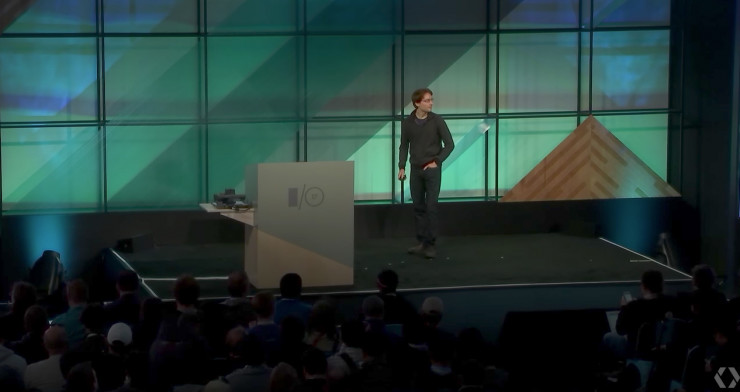雷锋网按：在上周的谷歌开发者大会 I/O 2017 的讲座中，Keras 之父 Francois Chollet 被请出来向全世界的机器学习开发者进行一场对 Keras 的综合介绍以及实战示例。说起来，这个子小小的男人不但是畅销书 《Deep learning with Python》的作者，更在 Kaggle 的数据科学家中世界排名第 17 位（最高），堪称是青年 AI 工程师中的翘楚。也因此，在开发出 Keras 之后被谷歌挖走为
TensorFlow 背书。

作为号称是 TensorFlow 最好用、对新手最友好的 API，一起来看看它的神通在哪里。

Francois Chollet：对许多使用场景而言，canned estimator 是相当不错的选择。但如果你要做的事并没有现成的 canned estimator，怎么办？如果需要写自己的定制模型呢？这时，就到了 Keras API 派上用场的时候。

什么是 Keras API？

简而言之，它就是一个用于创建 TensorFlow 模型的高级 API，你可以与它一起使用 estimator class 以及 experiment class。

众所周知，TensorFlow 的特点是非常低级的编程界面，你大多数时间花在矩阵、矢量乘法上。这使它成为一件非常强力的深度学习工具。但对于创建十分复杂先进的模型，这种操作方式说不上“理想”。

在谷歌，我们相信，未来深度学习将存在于每一个普通 IT 开发者的工具箱中，不再局限于机器学习专家。原因很简单：每个开发者都需要做出更智能的应用。

为了让这成为现实，我们需要降低深度学习的使用门槛，搞出每一个人都能用的工具，而不应该只有专家才能用深度学习解决问题。

我有一个问题想让大家思考：

如果设计一个没有任何束缚限制的深度学习界面，它应该是什么样的？

深度学习的核心理念很容易理解，它的实现也不应该复杂。对于模型核心部件，与其从头实现所有功能，应该让开发者利用现存部件，快速搭建数据处理流水线；就像乐高积木那样。这正是我们设计 Keras 的理念——成为“深度学习的乐高”。

Keras 能做什么？

下面，我来讲讲 Keras 都能做什么。

首先，我不建议把 Keras 看做是 codebase、框架或库，它只是个高级 API。它有不同的实现，最主要的当然是 TensorFlow，但还有基于其它平台的——目前有 Theano、MXnet 和 Java；对更多框架的支持正在路上。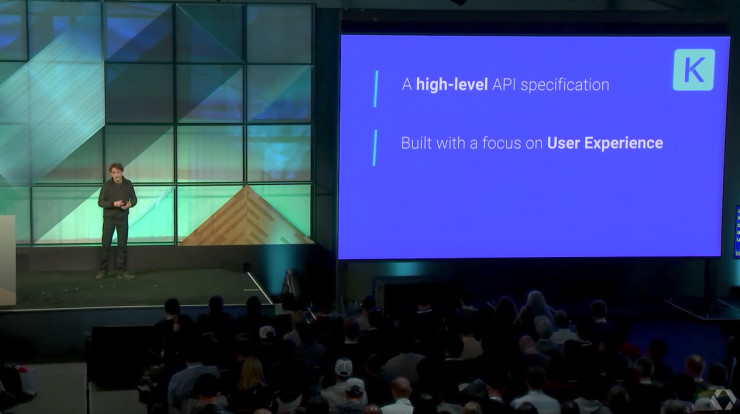有一个特点，是 Keras 区别于其他所有深度学习操作界面的地方，让它鹤立鸡群——那就是用户体验。说白了，Keras 的精髓就是它对用户体验的执着，让开发者用着更舒服、简化工作流。尤其在于提供易于使用的组件、符合直觉的推理、更好的报错方面。因而，Keras 降低了操作难度，降低使用者的认知负担。

当然，让深度学习变得更简单，同时意味着更多的人能上手。Keras 的终极目标，是让尽可能更多人接触、使用深度学习。

直到现在，Keras API 的 TensorFlow 实现，是以外部开源资源库的形式存在的。但现在，我们把 Keras API 直接整合入 TensorFlow 项目中，这样能与你的已有工作流无缝结合。至此，Keras 成为了 TensorFlow 内部的一个新模块：tf.keras，它包含完整的 Keras API。

“对于 TensorFlow 用户，这意味着你获得了一整套易于使用的深度学习组件，并能与你的工作流无缝整合。

对于 Keras 用户，这意味着一系列高级 TensorFlow 训练功能，比如分布式训练、分布式超参数优化。”

下面，我们一起来看看你的工作流会是什么样子。我会向大家展示一个简单但挺先进的例子。该例子中，我用 Keras API 定义模型，用 TensorFlow estimator 和 experiments 在分布式环境训练模型。

示例： 视频内容问答

这是一个视频问答问题。我们有一组 10 秒短视频组成的数据集，视频内容是人从事各种活动。一个深度学习模型将会观察这些视频的每一帧画面，进行理解，然后你可以用简短的自然语言问它视频内容。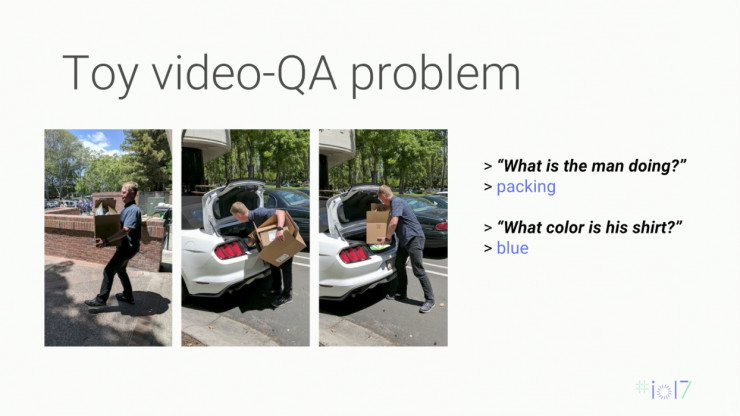本例子中，一个男人把纸板箱放进车的行李箱里。任务是回答这个人在做什么。模型会处理该视频和问题，试图在可能的答案中挑选出正确的那一个。这次，它的回答是“装货”。这个答案很有意思：如果仅仅看一帧画面，是得不出该结论的——这个人也有可能在卸货。所以，我们不仅要求模型能理解视频画面的内容，还要能理解每一帧画面的先后顺序。

放到三四年前，Keras 和 TensorFlow 诞生之前，这会是一个无比棘手的难题，全世界只有个位数的研究机构能处理。即便是一只由世界级专家学者、工程师组成的团队，也需要半年左右的时间来一点一点解决。而现在，所有具备基础 Python 编程技能的人都能借助工具处理该问题。我们这也是在使深度学习民主化。

下图便是我们的神经网络方案。它的结构可分为三个部分：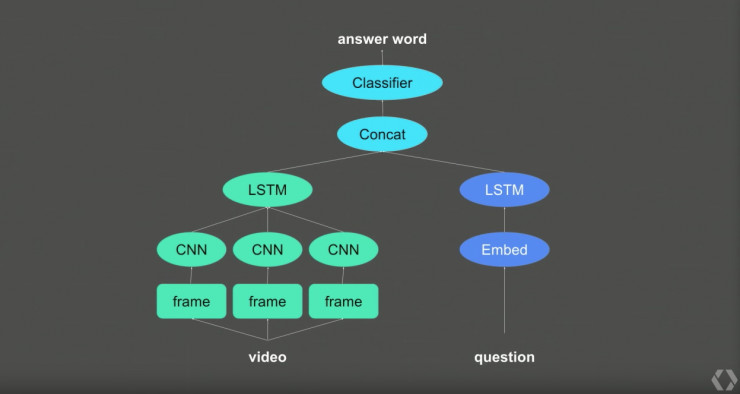首先，一个分支会导入视频输入，把它转化为对视频内容编码的矢量。另一个分支导入问题，也把它转化为矢量。现在，你可以把视频矢量和问题矢量连结起来，在它们之上添加一个分类器。该分类器的任务，是从一堆潜在回答中，选出正确的那一个。

第一步，是把视频输入矢量转化为张量。一个视频只是一组连续的画面帧，每一帧都是一个图像。对于图像处理，你要做的全部的事，就是运行一个 CNN。

每个 CNN，会从每帧画面提取一个矢量表示。最后所得到的，是对每帧画面进行编码的矢量序列。当遇到一个序列，你会做什么？当然是用序列处理模块—— LSTM 把它跑一遍。LSTM 会把序列简化为一个单一矢量，该矢量编码了视频的所有信息，包括每一帧画面、以及它们的顺序。

下一步，使用类似的过程来处理问句。它是一个由词语组成的序列，需要用内嵌模块把每个词语映射为一个词矢量。你就获得了一个词向量序列，再用另一个 LSTM 层来简化。

当视频、问题的矢量表示都有了以后，就可以把它们连接起来，在上面添加一个用于选择正确答案的分类器。

这就是深度学习的魔力：把复杂的输入，比如视频、图像、语言、声音变成矢量，变成几何空间中的不同的点——把了信息变成了几何空间中的点，这就是深度学习的本质。

而当完成之后，你就可以用线性代数来处理几何空间，捕捉到到有趣的映射模式。在上面的例子中，该模型就是在学习一个视频、问题空间到答案空间的映射。而执行的方式，是把不同的信息处理模块组合起来。这是一个十分自然的操作：对象是图像，就用图像处理模块 CNN；对象是序列，就用序列处理模块 LSTM；如果需要从一组候选中选择一个，就用分类器。

因而，创建深度学习模型，在概念上和拼乐高积木是很相似的，前者的实现也应该这么简单。这张图，就是对我们的模型在 Keras 上的直观结构。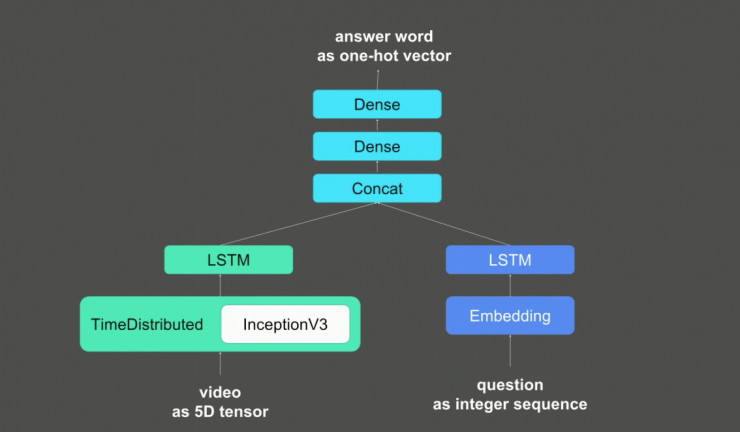我们用一个按时间分布的层，把 CNN 应用于由输入视频和张量组成的时间轴上的每一帧画面。然后把输入导入 LSTM 层，前者被简化为单一张量。InceptionV3 CNN 会内置预训练的权重，这一点很重要，因为以目前的视频输入，靠我们自己是无法学习到有趣的视觉特征的。我们需要利用现有的、在大型数据集上学习到的视觉特征。这个例子里是 ImageNet。在深度学习里，这是一个常见的举措，而 Keras 使它变得更方便。问题的编码更加简单。把词语序列导入内嵌层（embedding
layer），生成矢量序列，再用 LSTM 层简化为单一矢量。

代码演示

下面是视频编码机器人的完整代码，加起来只有几行，非常简洁。你从确认视频输入开始，高亮部分就是你的视频输入：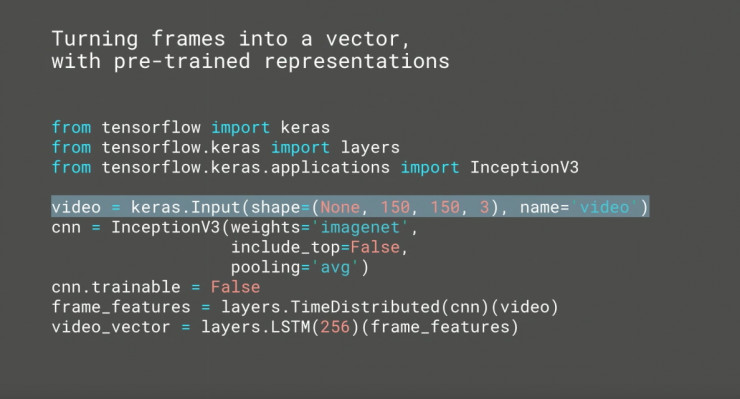这是一个由合理帧数组成的序列。“None”就是帧数，它没有被定义，你可以不同的 batch 进行修改。每一帧画面的分辨率是 150*150。下一步，仅用一行我们就定义了整个 InceptionV3 模型。它装满了从 ImageNet 得到的预训练权重。所有这些已经内置于 Keras 中，你不需要做任何多余操作，仅此一行代码足矣。代码并不包含顶层，因为并不相关，但在顶部加入了 pooling，使得我们能从每一帧抓取一个矢量。

下一步，CNN 被设置为不可训练，意味它的参数表示并不会在训练中更新。这一步很重要，因为该 CNN 已经有了非常不错的表示，没必要更改。再强调一遍，这是深度学习的常用操作，把封住不再改动的预训练模型添加入流水线。在 Keras 中，这项操作变得十分简便。有了不再变动的 CNN 之后，我们用一个时间分配层（time distributed layer），把它在视频输入的时间轴上均衡分配。这样做的结果，是得到所有帧的张量，再导入
LSTM 层得到单一矢量。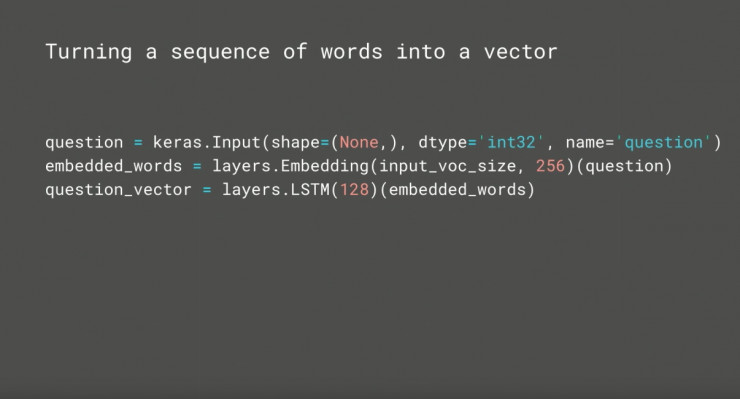如上图，问题处理就更加简单。最终的问题输入，被处理为整数序列。为什么是整数呢？每一个整数，都会用某些词汇映射到一个矢量。随后把整数序列导入嵌入层，这会把每个整数映射到一个矢量上。这些训练过的嵌入是模型的一部分。再把矢量序列导入 LSTM，简化为单一矢量。

这里有一个有意思的地方。通常使用 LSTM 的时候，有许多东西需要考虑、许多套路需要参考。但在这里，除了设置输入单位的数量，我们并没有做任何其他操作配置 LSTM 层——所有“最佳套路”，都已经成为 Keras 的默认设置。这是 Keras 的一大特点，已知的最佳方案被用于默认设置。对于开发者，这意味着模型直接就能用，不需要对所有参数都进行调参。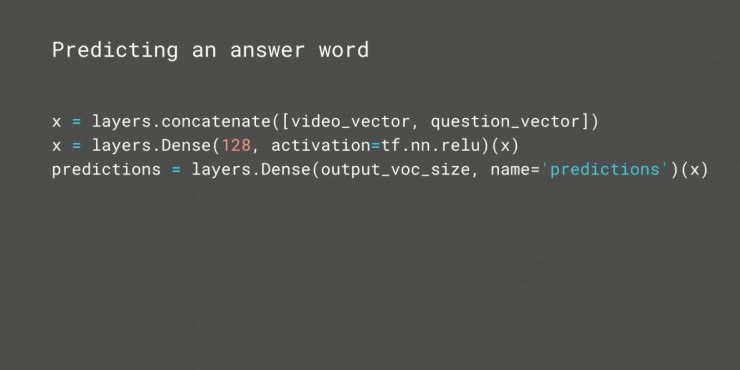在完成对视频、问题的编码之后，你只需要用 concate up 把它们转化为单一矢量，然后在顶端加入两个密集层，它们会从备选词汇中选出一个作为答案。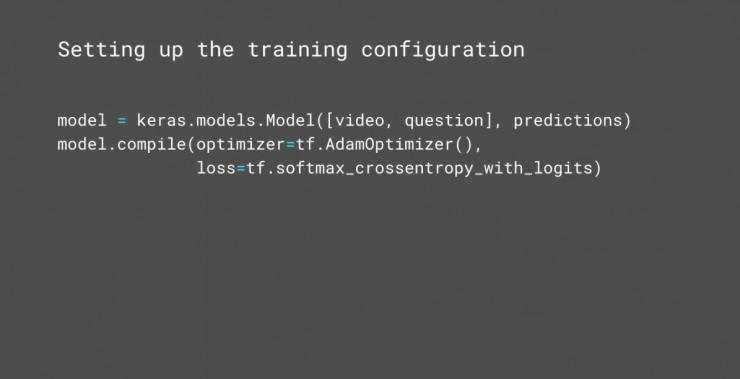下一步，使用输入和输出初始化 Keras 模型，本质上它是一个神经网络各层的图（a graph of layers）的容器。然后要确定训练设置，比如优化器、Adam 优化器和损失函数。到现在一切都很简单，我们已经定义了模型和训练设置。下面是在分布式环境训练模型，或许在 Cloud ML 上。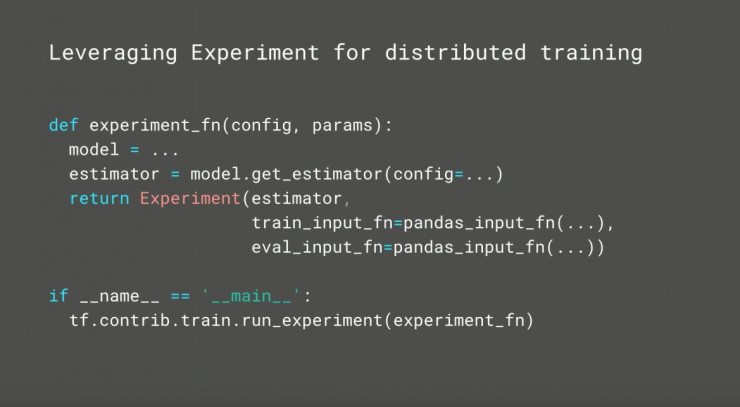只用几行代码，你就可以用 TensorFlow Estimator 和 Experiment 类训练模型。所有需要你做的事，仅仅是写 experiment 函数，用内置的 get_estimator 方法在其中定义模型，并用模型来初始化 Estimator。有了 estimator 之后，再用它创建 Experiment，在其中你确认输入数据。

仅仅用几行非常直观、具有高度可读性的 Python 代码就可以实现，我们就定义了一个相当先进的模型、在分布式环境训练它，来解决视频问答难题。而这在几年前是完全难以想象的。

到这里，你应该已经看到，像 Keras 这样的 API 是如何推动 AI 民主化。这借助两个东西实现：

其中一个，当然是 Keras API。为在 TensorFlow 中定义模型提供了易于使用、功能强大的工具。而且，每一层都有非常优秀的默认设置，让模型可以直接运行。

另外一个，则是全新的高级 TensorFlow 训练 API：Estimator 和 Experiment。

把它们结合到一起，使得开发者们能够以相当小的时间、经历代价处理任何深度学习难题。展开全文keras 机器学习 Tensorflow
• 前言 连滚带爬的摸索了一周，网上的教程参差不齐，都是在讲一大堆概念，而没有动手的教程。就算是动手的教程，也大都版本太旧，而且是在理想状态下的试验，不符合每个人的需求。最后经过摸索，终于跑通了！...
• Keras上使用 我们已经将 tf.distribute.Strategy 集成到 tf.keras 中。tf.keras 是一个构建和训练模型的高级API。通过集成到 tf.keras 后端, 用Keras训练框架写的程序可以无缝进行分布式训练。 您需要对代码中进行...
• Keras作为tensorflow的精简接口 文章信息 本文地址：https://blog.keras.io/keras-as-a-simplified-interface-to-tensorflow-tutorial.html 本文作者：Francois Chollet 使用Keras作为TensorFlow工作流的一...
• Horovod是基于Ring-AllReduce方法的深度分布式学习插件，以支持多种流行架构包括TensorFlow、Keras、PyTorch等。这样平台开发者只需要为Horovod进行配置，而不是对每个架构有不同的配置方法。 Ring-AllReduce方法是...
• keras与torch7的使用非常相似，是最近才火起来的深度学习开源库，底层是用了theano。keras可以说是python版的torch7，对于快速构建CNN模型非常方便。同时也包含了一些最新文献的算法，比如Batch Noramlize，文档教程...
• ## Keras

千次阅读 2018-08-11 14:45:57
Keras是由纯python编写的基于theano/tensorflow的深度学习框架。Keras是一个高层神经网络API，支持快速实验，能够把你的idea迅速转换为结果，如果有如下需求，可以优先选择Keras： a）简易和快速的原型设计（keras...
• Keras是一种高度模块化，使用简单上手快，合适深度学习初学者使用的深度学习框架。Keras由纯Python编写而成并以Tensorflow、Theano以及CNTK为后端。Keras为支持实验而生，能够把你的idea迅速转换为结果。 对于深度...keras python tensorflow
• ## 查看keras版本

万次阅读 2018-03-30 10:23:40
打开终端依次输入以下两个命令。我的默认是2.1.1版本的python print(keras.__version__)
• ## Keras和TensorFlow的关系和区别

万次阅读 多人点赞 2017-12-21 18:40:54
TensorFlow和theano以及Keras都是深度学习框架，TensorFlow和theano比较灵活，也比较难学，它们其实就是一个微分器 Keras其实就是TensorFlow和Keras的接口（Keras作为前端，TensorFlow或theano作为后端），它也很...
• pip install --upgrade keras==2.1.0 升级到指定版本pip install keras==2.0.9安装指定版本
• 安装 keras-contribpip install git+https://www.github.com/keras-team/keras-contrib.git[参考文献]https://blog.csdn.net/qq_16912257/article/details/78969966
• 因为需要安装Anaconda+python3.6+tensorflow+keras的环境，在进行到最后一步keras安装的时候出现了 No module named ‘keras’ 试了各种办法都不成功，仔细回想了一下，是在之前安装了python2.7、python3.5、...
• ## Keras安装与测试

万次阅读 2018-08-19 12:22:55
Keras是高度封装的包，适合初学者学习深度学习网络框架，比如我这个小白，一切都在尝试中，每天都在安装各种库各种API！！！ Keras 安装： 环境 anconda（含pip，python3.6） 本人是在cmd中使用pip安装的keras，...keras
• Keras版本 Tensorflow 2.1 Tensorflow 2.0 Tensorflow 1.15 Keras 2.3.1 Tensorflow 1.14 Keras 2.2.5 Tensorflow 1.13 Tensorflow 1.12 Tensorflow 1.11 Keras 2.2.4 Tensorflow 1.10 Tensorflow 1.9 ...tensorflow
...